# A Mixed Constraint Problem[Page A-21]

So far we have discussed maximization problems with all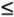constraints and minimization problems with all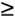constraints. However, we have yet to solve a problem with a mixture of,, and = constraints. Furthermore, we have not yet looked at a maximization problem with aconstraint. The following is a maximization problem with,, and = constraints.

A mixed constraint problem includes a combination of, =, andconstraints.

A leather shop makes custom-designed , hand-tooled briefcases and luggage. The shop makes a \$400 profit from each briefcase and a \$200 profit from each piece of luggage. (The profit for briefcases is higher because briefcases require more hand tooling.) The shop has a contract to provide a store with exactly 30 items per month. A tannery supplies the shop with at least 80 square yards of leather per month. The shop must use at least this amount but can order more. Each briefcase requires 2 square yards of leather; each piece of luggage requires 8 square yards of leather. From past performance, the shop owners know they cannot make more than 20 briefcases per month. They want to know the number of briefcases and pieces of luggage to produce in order to maximize profit.

This problem is formulated as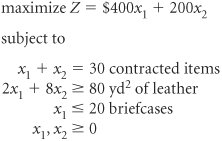where x 1 = briefcases and x 2 = pieces of luggage.

The first step in the simplex method is to transform the inequalities into equations. The first constraint for the contracted items is already an equation; therefore, it is not necessary to add a slack variable. There can be no slack in the contract with the store because exactly 30 items must be delivered. Even though this equation already appears to be in the necessary form for simplex solution, let us test it at the origin to see if it meets the starting requirements.

 x 1 + x 2 = 30 0 + 0 = 30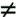30

Because zero does not equal 30, the constraint is not feasible in this form. Recall that aconstraint did not work at the origin either in an earlier problem. Therefore, an artificial variable was added. The same thing can be done here.

x 1 + x 2 + A 1 = 30

Now at the origin, where x 1 = 0 and x 2 = 0,

 0 + 0 + A 1 = 30 A 1 = 30

An artificial variable is added to an equality (=) constraint for standard form.

Any time a constraint is initially an equation, an artificial variable is added. However, the artificial variable cannot be assigned a value of M in the objective function of a maximization problem. Because the objective is to maximize profit, a positive M value would represent a large positive profit that would definitely end up in the final solution. Because an artificial variable has no real meaning and is inserted into the model merely to create an initial solution at the origin, its existence in the final solution would render the solution meaningless. To prevent this from happening, we must give the artificial variable a large cost contribution, or M .

[Page A-22]

The constraint for leather is ainequality. It is converted to equation form by subtracting a surplus variable and adding an artificial variable:

2 x 1 + 8x 2 s 1 + A 2 = 80

An artificial variable in a maximization problem is given a large cost contribution to drive it out of the problem.

As in the equality constraint, the artificial variable in this constraint must be assigned an objective function coefficient of M .

The final constraint is ainequality and is transformed by adding a slack variable:

x 1 + s 2 = 20

The completely transformed linear programming problem is as follows :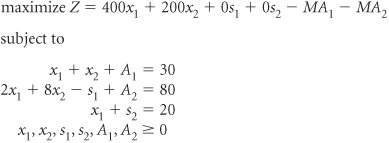The initial simplex tableau for this model is shown in Table A-21. Notice that the basic solution variables are a mix of artificial and slack variables . Note also that the third-row quotient for determining the pivot row (20 ·0) is an undefined value, or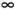. Therefore, this row would never be considered as a candidate for the pivot row. The second, third, and optimal tableaus for this problem are shown in Tables A-22, A-23, and A-24.

Basic Variables

Quantity

400

200

M

M

c j

x 1

x 2

s 1

s 2

A 1

A 2

M

A 1

30

1

1

1

M

A 2

80

2

8

1

1

s 2

20

1

1

z j

110 M

3 M

9 M

M

M

M

c j z j

400 + 3 M

200 + 9 M

M

Basic Variables

Quantity

400

200

M

c j

x 1

x 2

s 1

s 2

A 1

M

A 1

20

3/4

1/8

1

200

x 2

10

1/4

1

1/8

s 2

20

1

1

z j

2,000 20 M

50 3 M /4

200

25 M /8

M

c j z j

350 + 3 M /4

25 + M /8

[Page A-23]

Basic Variables

Quantity

400

200

M

c j

x 1

x 2

s 1

s 2

A 1

M

A 1

5

1/8

3/4

1

200

x 2

5

1

1/8

1/4

400

x 1

20

1

1

z j

9,000 5 M

400

200

25 M /8

350 + 3 M /4

M

c j z j

25 + M /8

350 3 M /4

##### Table A-24. The Optimal Simplex Tableau

Basic Variables

Quantity

400

500

c j

x 1

x 2

s 1

s 2

s 1

40

1

6

200

x 2

10

1

1

400

x 1

20

1

1

z j

10,000

400

200

200

c j z j

200

The solution for the leather shop problem is (see Table A-24):

x 1 = 20 briefcases

x 2 = 10 pieces of luggage

s 1 = 40 extra yd 2 of leather

Z = \$10,000 profit per month

It is now possible to summarize a set of rules for transforming all three types of model constraints.

Objective Function Coefficient

Constraint

Adjustment

M AXIMIZATION

M INIMIZATIONAdd a slack variable

=

Add an artificial variable

M

MSubtract a surplus variable

and add an artificial variable

M

MIntroduction to Management Science (10th Edition)
ISBN: 0136064361
EAN: 2147483647
Year: 2006
Pages: 358

Similar book on Amazon

flylib.com © 2008-2017.
If you may any questions please contact us: flylib@qtcs.net